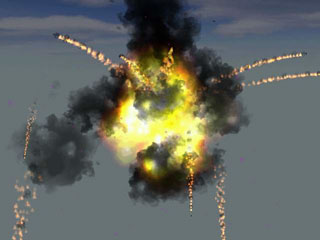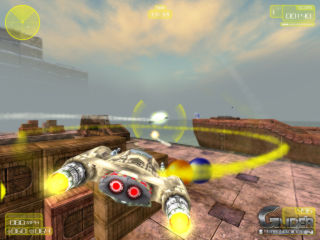## effect_layer (function, var number, VECTOR* pos, VECTOR* vel)

Creates a swarm of little moving bitmap particles, for rocket trails, explosions, laser beams, photon torpedos, rain, snowstorms, tornados or the like. The pos vector gives the starting position of the swarm, number gives the number of particles, vel gives either the initial speed or the beam length, and the function defines the behavior of each particle. The function is called permanently during the lifespan of each single particle and can change the position, speed, color, size, transparency, and bitmap of the particle, in a similar way as with normal entities. It can even create new 'children' particles. This gives the flexibility to use particle effects of every description.Particle effect Beam effect

### Parameters:

 function Particle function; runs every frame. number Number of particles to create. pos Position of the particle emitter. vel Initial speed vector or beam length.

### Remarks:

• The particles are not created immediately after calling this function, but at the start of the next render cycle.
• When executed by a client in a multiplayer environment, effect_local creates a local particle effect that is only visible on the client. Executed on the server, effect creates a global particle effect, which is then automatically sent to and executed on all clients. In a single player environment, both instructions behave identically.
• A8 The effect_layer function creates the effect outside the level, just like layered entities. This way effects can be placed on panels or follow the mouse pointer. Particles created with effect_layer are rendered on the media_layer, and use the view settings from the camera view.
• You can have many thousands of particles, so the particle function should be as fast and as short as possible. wait() instructions can not be used in a particle function. Local variables can be used, but are not preserved between frames. For storing variables between frames, particle skills can be used.
• Particles are optimized for speed, thus their rendering is kept as simple as possible. They are two dimensional and have no angles, no collision detection, and only primitive lighting, sorting, and clipping. If you need angles or better rendering, or if the particles are large, better use effect_sprites for creating a swarm of sprites. If you need collision detection, the fastest way is to directly calculate the distance to a collision plane - ideally an axis aligned plane - within the particle function. c_trace can also be used, but would make the effect slow.
• Particles are rendered at the end of the frame cycle after all other objects. This means that transparent particles appear above other transparent objects. For avoiding that you can give the particles a non transparent, overlay bitmap. If you need to sort transparent particles, use effect_sprites for creating a swarm of sprites instead.
• If effect_local was called by an entity, the particle functions can access this entity through the you pointer. This way, the particle effect can use entity parameters.
• For keeping the particle number independent of the frame rate on continuous particle generation, multiply the number of generated particles with time_step (see example).
•  P  The Pro Edition uses instancing (a method supported by new graphics cards to render many copies of the same object) for creating thousands of particles with one single drawing function. This way up to ten times more particles can be rendered than with the lower editions. This feature can be switched off through the particle_mode variable.
• The total number of particles can be limited through the max_particles variable.
• A8 Particle functions can be temporarily suspended with freeze_mode.

Fast

### Demo (lite-C):

```// helper function: sets the vector to random direction and length
function vec_randomize (VECTOR* vec, var range)
{
vec_set(vec,vector(random(1)-0.5,random(1)-0.5,random(1)-0.5));
vec_normalize(vec,random(range));
}

// helper function: fades out a particle
{
p.alpha -= p.skill_a*time_step;
if (p.alpha <= 0) p.lifespan = 0;
}

function p_fountain(PARTICLE* p)
{
VECTOR vTemp;
vec_randomize(vTemp,2);
vec_set(p.blue,vector(random(255),random(255),255));
set(p, MOVE | BRIGHT | TRANSLUCENT);
p.alpha = 100;
p.size = 2;
p.gravity = 0.2;
p.skill_a = 3; // fade factor
}

function main()
{
video_window(NULL,NULL,0,"Particle demo");
vec_set(camera.x,vector(-150,0,50));
vec_set(sky_color,vector(50,0,0)); // dark blue

while(1)
{
effect(p_fountain,maxv(1,40*time_step),vector(0,0,0),vector(0,0,5));
wait(1);
}
}```